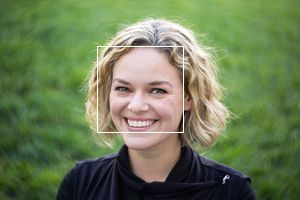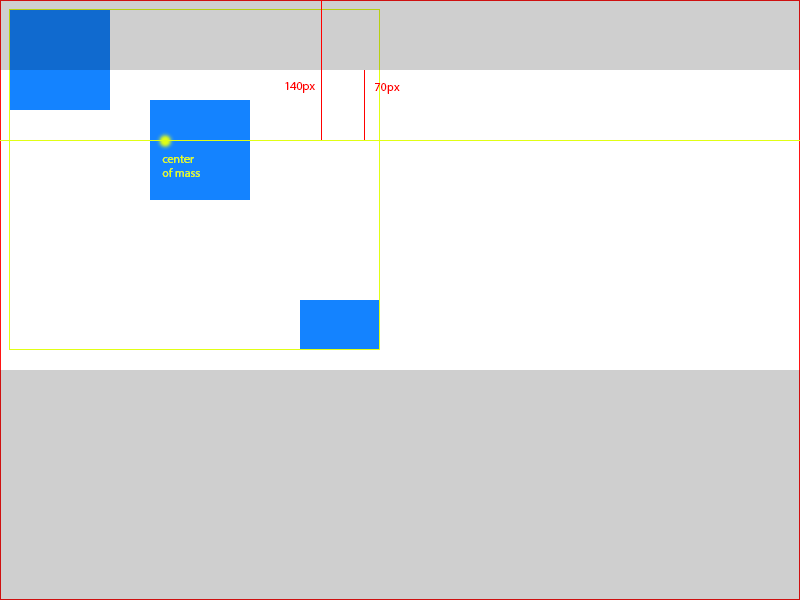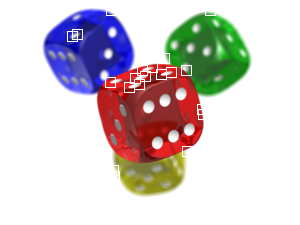# Detection Algorithms¶

If the smart mode of thumbor has been specified in the uri (by the /smart portion of it), thumbor will use it’s smart detectors to find focal points.

thumbor comes pre-packaged with two focal-point detection algorithms: facial and feature. First it tries to identify faces and if it can’t find any, it tries to identify features (more on that below).

## Facial Detection¶

For instructions on how to get facial detection coordinates see Metadata Endpoint .

thumbor uses OpenCV (http://opencv.org) to detect faces. OpenCV returns the rectangle coordinates for the faces it identifies. Thumbor supports changing the file that OpenCV uses for identifying faces. We can see the algorithm in action below:

Original imageRed rectangles are the areas identified as facesAfter retrieving these squares from OpenCV, thumbor calculates the center of mass of the image using weighted average.

Consider that OpenCV returned 3 squares at (10, 10, 100, 100), (150, 100, 100, 100), (300, 300, 80, 50), being (x, y, width, height), as such:In order to find the center of mass for all the faces, we must first find the center and weight of each rectangle. We define weight in this scenario as the area of the rectangle.

So, for the faces in our example (*x*,*y* being the coordinates of the rectangle’s center and *z* the rectangle weight):

Face 1 - (x = (10 + 100) / 2 = 55), (y = (10 + 100) / 2 = 55), (z = 100 * 100 = 10000)

Face 2 - (x = (150 + 100) / 2 = 125), (y = (100 + 100) / 2 = 100), (z = 100 * 100 = 10000)

Face 3 - (x = (300 + 80) / 2 = 190), (y = (300 + 50) / 2 = 175), (z = 80 * 50 = 4000)

In order to find the center of mass we’ll do a weighted average of the X and Y coordinates of the faces using:

Horizontal Axis - X (((55 * 10000) + (125 * 10000) + (190 * 4000)) / 24000 = 106)

Vertical Axis - Y (((55 * 10000) + (100 * 10000) + (175 * 4000)) / 24000 = 93).

So for the faces found by OpenCV in that image we have the center of mass of the picture being 106x93.

### Using Focal Points for Cropping¶

After finding the center of mass we can use it as the focal point for cropping. Given an image of 800x600 and a focal point at 106x93, we need to determine the percentage that needs to be cropped from the top, bottom, left and right sides of the image.

To determine the percentage we use simple math to figure how far from the top and the left side the center of mass is:

From the left - (106 / 800 * 100 = 13.25%)

From the top - (93 / 600 * 100 = 15.50%)

Using the same example from the Crop and Resize Algorithms page, we need to crop 300px out of the height of the image. In possession of the percentages of crop above, we can calculate how much we need to crop out of the top and bottom with:

Top - (300 * 0.155 ~= 46)

Bottom - just subtract top (46px) from the amount of crop (300px) - (300 - 46 = 254)

So, now we now we have to remove 46px out of the top of the picture and 254px out of the bottom of the picture. In an 800x600 picture, that means cropping from (0, 46) to (800, 346), resulting in an 800x300px image.

Assuming we would crop 300px horizontally, the cropping would be:

Left - (300 * 0.135 ~= 40)

Right - just subtract left (40px) from the amount of crop (300px) - (300 - 40 = 260)

In an image of 800x600, that means cropping from (40, 0) to (540, 600), resulting in a 500x600px image. This would not be the case for this image, though.

## Feature Detection¶

If no faces are found in the picture, we still try to find important features in the image, provided by the Good Features to Track Algorithm in OpenCV (http://bit.ly/evAU95).

According to OpenCV documentation, this algorithm finds “important” corners in the image. It then returns a list of (x, y) values.

We can see the detection taking place in the following images:The points identified by the good features algorithm:The cropping based in these features is analogous to the face one, except that all points have a weight of *1.0* and are already their centers.

Let’s consider that we found 3 feature points: 10x15, 30x40, 25x60. To find the center of mass we would do ((10 + 30 + 25) / 3 ~= 22) to find the horizontal component and ((15 + 40 + 60) / 3 ~= 39) for the vertical one. This means that our center of mass in this scenario is *22x39*.

Given an image of 800x600 and a center of mass of 22x39, let’s find the left and top percentages:

From the left - (22 / 800 * 100 = 2.75%)

From the top - (93 / 600 * 100 = 6.50%)

Assuming we are cropping 300px of the height, we’ll crop top and bottom according to:

Top - (300 * 0.0275 ~= 9)

Bottom - just subtract top (9px) from the amount of crop (300px) - (300 - 9 = 291)

In an image of 800x600, that means cropping from (0, 9) to (800, 309), resulting in a 800x300px image.

If we were cropping 300px of the width instead, we would crop left and right according to:

Left - (300 * 0.065 ~= 20)

Right - just subtract left (20px) from the amount of crop (300px) - (300 - 20 = 280)

In an image of 800x600, that means cropping from (20, 0) to (520, 600), resulting in a 500x600px image.